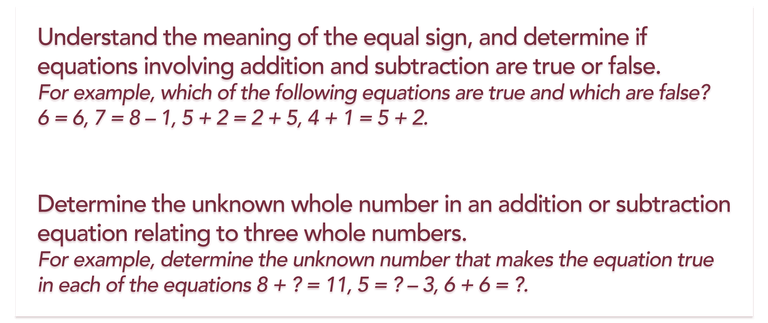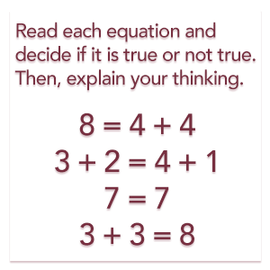## All things being equal, the equals sign deserves more attention.

by Rob Schoen | October 5, 2017
Equals Sign

The equals sign has a deep, almost sacred, meaning in mathematics. Despite the importance of = as a relational operator in mathematics that is used at all levels K–20 (and beyond), many elementary, secondary, and even post-secondary mathematics students have a limited understanding of the intended meaning of that symbol in mathematics.

For seven years and counting, the mathematics curriculum standards in Florida (and almost every state in the United States) have set explicit learning goals for student understanding of the equals sign. In most states, the following two content standards are in place for first grade:Two first-grade content standards in the Florida curriculum standards for mathematics - MAFS.1.OA.4.7 and MAFS.1.OA.4.8.

The writers of the standards, in my view, did students a great service by translating research on student thinking related to the equals sign into policy.

Many teachers look at these standards and ignore them or think they’re trivial. Or, maybe they interpret the intent of the standard to be focused on students knowing basic facts rather than the meaning of the equals sign as a relational symbol. As many other teachers know, those teachers are overlooking an important component of student understanding.

Several years ago, I found myself in a fortunate position to lead an amazing team of teacher-researchers working to develop tasks and rubrics for (commonly called MFAS). Our goal was to develop at least four good mathematics problems, or tasks, aligned with each of the content standards at each grade level and designed to provide teachers with insight into student understanding that could inform their instructional decisions. Each task had a corresponding, task-specific rubric with four levels. Over several years, we conducted thousands of student interviews in the process of piloting and refining the tasks and rubrics. We had a group of partner teachers in four school districts in Florida who kindly allowed us to interview their students. In exchange, we would tell the teachers what we learned about their students.A sample set of mathematical statements used in our interviews with first-graders.

In the development of tasks and rubrics for the first-grade equals-sign standards, we interviewed dozens of first-graders in four school districts. We had created a set of mathematical statements in the form of simple equations (some true, some not true) and asked first-grade students to read each equation and tell us whether or not the equation was true. While almost all of the students performed the operations on the numbers accurately and used vocabulary words such as equation correctly, none of the students answered the true-false questions about these equations correctly.

The first-grade students we interviewed were very consistent in their explanations for why they decided the statement 7 = 7 was false, or not correct. They explained that the equation did not have a plus or minus sign, so it wasn’t correct. They told us that 8 = 4 + 4 is not correct, because the plus sign was on the wrong side of the equals sign. They knew vocabulary terms such as equation, subtraction, plus, add, etc. They also knew how to evaluate expressions such as 4 + 4. Across dozens of first-grade students, they were almost 100% consistent in saying 7 = 7 was not correct, because there was no addition or subtraction symbol and that 8 = 4 + 4 was not correct, because the plus sign was on the wrong side of the equals sign.

We are certain that teachers never told their students that 6 = 6 is a false statement! They probably never even said that it was an incorrect way to write an equation. It seems most plausible that the first-graders had simply never seen equations written in that form. Rather, they probably had only seen them in the form a + b = c or  b = c. Because they had never been exposed to equations in other forms, they assumed those other forms to be incorrect.

This was a problem for us, because we weren’t getting examples of what students would say if they were in the higher levels of the rubrics, and we wanted to have real examples of what students at each of the four levels of the rubric would do.

Fortunately for us, the partner teachers were serious about teaching mathematics! When we reported what we learned about student understanding of the equals sign to their teachers, the teachers were often surprised. As soon as they learned about this issue, they went right to work on trying to improve and expand their students’ understanding of the intended meaning of =. Many teachers asked for recommendations for how to address this pervasive problem.

Here are three recommendations that came from those conversation with teachers. While we think these recommendations apply to first-grade students, we also think they apply to kindergarten students, second-grade students, and even students at the secondary and post-secondary level.

1. Discuss the mathematical meaning of the equals sign with students. Don’t just use the symbol and assume students will grasp the intended meaning of = in mathematics. Talk explicitly about its intended meaning with your students, and reinforce it many times throughout the year.
2. Expose students to non-standard forms of equations (e.g., 8 = 4 + 4) frequently, and state explicitly when those expressions of mathematical ideas can be perfectly fine and correct to use in mathematics.
3. Introduce = as a relational symbol rather than as a command. In other words, try to create a closer association between =, <, and > in your students’ minds than there is between = and other operators such as + or –.

After incorporating these recommendations, our partner teachers reported that some students’ understanding of the equals sign changed quickly (and for the better). Other students’ ideas were more resistant to change. Like many other topics in mathematics (See Place Value), students can appear to fully understand the intended meaning of the equals sign one day and then appear to not have that same knowledge later.

***

We’ll have more blog posts in this series about the equals sign in the coming weeks. Each writer will share their unique perspective on the topic of the equals sign. I hope you will join the conversation to share your experiences and perspective on this topic. If you want, you can even write a guest post (email me for details). We invite you to share your own unique experiences in the comments so that we can work together to address this interesting problem of mathematics teaching.

 This task and the associated rubrics, including videos of student responses, can be found here. Several hundred other tasks and task-specific rubrics were created through the MFAS project and available free of charge through CPALMS.